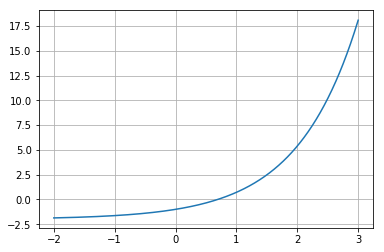# Convergence of Newton's Method¶

In :
import numpy as np
import matplotlib.pyplot as pt

In :
def f(x):
return np.exp(x) - 2

In :
xgrid = np.linspace(-2, 3, 1000)
pt.grid()
pt.plot(xgrid, f(xgrid))

Out:
[<matplotlib.lines.Line2D at 0x7fca94ed4048>]What's the true solution of $f(x)=0$?

In :
xtrue = np.log(2)
print(xtrue)
print(f(xtrue))

0.6931471805599453
0.0


Now let's run Newton's method and keep track of the errors:

In :
errors = []
x = 2
xbefore = 3


At each iteration, print the current guess and the error.

In :
slope = (f(x)-f(xbefore))/(x-xbefore)

xbefore = x
x = x - f(x)/slope
print(x)
errors.append(abs(x-xtrue))
print(errors[-1])

nan
nan

/home/andreas/src/env-3.7/lib/python3.7/site-packages/ipykernel_launcher.py:2: RuntimeWarning: invalid value encountered in double_scalars


In :
for err in errors:
print(err)

0.8824000774932711
0.411823511031029
0.14748204487642924
0.027685962340313952
0.0019826842906380815
2.731067240058227e-05
2.706515089823114e-08
3.695932448977146e-13
1.1102230246251565e-16
1.1102230246251565e-16
nan

• Do you have a hypothesis about the order of convergence?
In :
# Does not quite double the number of digits each round--unclear.


Let's check:

In :
for i in range(len(errors)-1):
print(errors[i+1]/errors[i]**1.618)

0.5042249099646489
0.6196358421422753
0.6126884285565872
0.6571696439293109
0.6447273943575542
0.6552765724242319
0.6487597717813403
14482.140529886734
7243300082.988035
nan